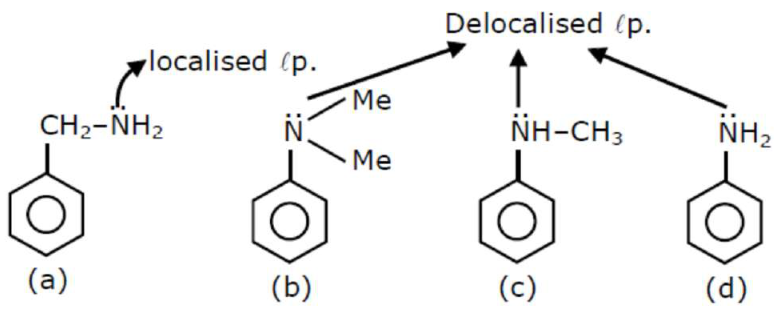# Choose the correct order of basic nature of the above amines.Question:

A. Phenyl methanamine

B. $N$, N-Dimethylaniline

C. N-Methyl aniline

D. Benzenamine

Choose the correct order of basic nature of the above amines.

1.  $D>C>B>A$

2. $D>B>C>A$

3.  $A>C>B>D$

4. $A>B>C>D$

Correct Option: , 4

Solution: